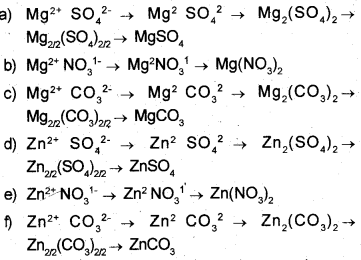# Kerala Syllabus 9th Standard Chemistry Solutions Chapter 5 Acids, Bases, Salts

You can Download Wave Motion Questions and Answers, Summary, Activity, Notes, Kerala Syllabus 9th Standard Chemistry Solutions Part 2 Chapter 5 help you to revise complete Syllabus and score more marks in your examinations.

## Kerala State Syllabus 9th Standard Chemistry Solutions Chapter 5 Acids, Bases, Salts

### Acids, Bases, Salts Textual Questions and Answers

Learning Activities

classify these salts as acidic, basic, or neutral Question 1.
What are the methods used to identify acids and alkalies
Using indicators like litmus, phenolphthalein and methyl orange.Question 2.
Find the characteristics of the substances given in the table below using litmus papers.

 Substance Change in the color of litmus Characteristics Vinegar Lime water Soap solution Hydrochloric acid

 Substance Change in the color of litmus Characteristics Vinegar Blue turns red .Acidic Lime water Red litmus turns blue Alkaline Soap solution Red litmus. turns blue Alkaline Hydrochloric acid Blue litmus turns red Acidic

Question 3.
Take a small piece of zinc in a test tube as shown in the figure. Add 2ml of dilute hydrochloric acid using a dropper. Show a burning match stick at the mouth of the test tube. Record the observation.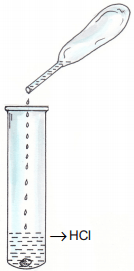Burn with a ‘pop’ sound

Question 4.
What would be the reason?
Hydrogen gas liberated burns

Question 5.
Now complete the chemical equation of this reaction
Zn + 2HCl → ZnCl2 + …………..
Zn + 2HCl → ZnCl2 + H2
Acids react with reactive metals to form hydrogen gas

Question 6.
Take some calcium carbonate (marble pieces) in a boiling tube as shown in figure. Add dilute hydro¬chloric acid to it through a thistle funnel. Pass the evolving gas through clear lime water taken in a test tube.Which is the gas that comes out through the delivery tube?
Carbon dioxide

Ques 7.
What is your observation when this gas is passed through clear lime water?
LimeWater turns milky
When acids react with carbonates, carbondioxide(CO2) gas is liberated.Question 8.
From the characteristics given below, find out those suitable for acids and put (✓) Mark.
1. Have alkaline taste.
2. Turn blue litmus red
3. React with carbonates to form carbon dioxide gas
4. Soapy to touch
5. Liberate hydrogen on reaction with highly reactive metals like Mg and Zn
6. Have sour taste
7. Turns red litmus blue

1. Have alkaline taste.
2. Turn blue litmus red (✓)
3. React with carbonates to form carbon dioxide gas (✓)
4. Soapy to touch
5. Liberate hydrogen on reaction with highly reactive metals like Mg and Zn (✓)
6. Have sour taste (✓)
7. Turns red litmus blue

Question 9.
The name of some familiar acids and their chemical formulae are given in the table below. Complete the table.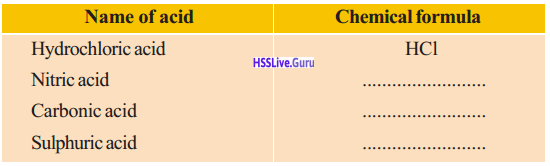Name of acid Chemical formula Hydrochloric acid HCI Nitric acid HNO3 Carbonic acid H2CO3 Sulphuric acid H2SO4

Question 10.
Which component is responsible for the common properties of acids
H

Question 11.
Chemical equations showing the formation of oppositely charged ions when hydrochloric acid (HCI) and nitric acid (HN03) dissolve in water are given.
HCI → H++Cl
HNO3 → H++ NO
a) Which are the ions present in HCl solution?
b) Which are the ions present in HNO3 solution
c) Which is the ion common to both?
a) H+ and Cl
b) H+ and NO3
c) H+

Question 12.
What is acids?
Acids are substances which can increase the concentration of hydrogen (H+) ions in an aqueous solution.

Question 13.
List the acids that are present in lime juice, curd, tamarind, vinegar.
Lime juice — Citric acid
Curd — Lactic acid
Tamarind — Tartaric acid
Vinegar — Acetic acid

Question 14.
Write the chemical equation for the ionization of HCl.
HCl → H++Cl

Ques 15.
How many hydrogen ions are released when one molecule of HCI is ionized?
one H+

Question 16.
What is the basicity of an acid?
The number of hydrogen ions that can be donated by one molecule of an acid is its basicity.
If the basicity is 1, it is called monobasic acid.

Question 17.
Write the ionization equation of nitric acid (HNO3) and find its basicity.
HNO3 → H+ +NO3
Basicity -1

Question 18.
Write the chemical equation for the ionization of the sulphuric acid (H2S04)
H2SO4 → H+ + HSO4 (bisulphate ion)
HSO4 → H+ + SO24 (sulphate ion)

Question 19.
How many hydrogen ions are released when one molecule of H2SO4 gets ionized? What is its basicity? ’
Two H+ ions basicity = 2
It the basicity of an acid is 2,
it is said to be a dibasic acid.

Question 20.
Complete the ionization equation of phosphoric acid (H3PO4).
H3PO4 → ………… + PO34(Phosphate ion)
H3PO4 → 3H+ + PO34 (Phosphate ion)

Question 21.
What is the basicity of H3PO4?
3
If the basicity is 3,
the acid is called a tribasic acid.

Ques 22.
The chemical formulae of some acids are given in the box. Pick out monobasic and dibasic acids.
H2CO3, HNO3, H3PO4, H2SO3, HCI, H2SO4
Monobasic: HNO3, HCI
Dibasic: H2CO3, H2SO3, H2SO4

Question 23.
How do you make soda water? Write the equation of this reaction.
CO2 is dissolved in water to make soda water.
H2O + CO2 → H2CO3 (Carbonic acid)

Question 24.
Complete the equation of dissolution of SO2 in water.
………… + ………….. → H2SO3 (sulfurous acid)
SO2 + H2O → H2SO3 (sulfurous acid)
CO2, SO2 and NO2 are non-metallic oxides. Generally, compounds formed by the reaction of non-metallic oxides with water are acidic.Question 25.
What is acid rain?
In industrial areas and townships, the chances of air pollution are very high. In such regions, gases like SO2 and NO2 reach the atmosphere in larger amounts. These gases dissolve in rainwater and reach the soil as acids. This is known as ‘acid rain’.

Question 26.
What are the environmental problems caused by acid rain?

• Plants lose their ability to produce carbohydrates through photosynthesis as their leaves are de- strayed.
• Severe acid rain destroys the greenery of a region.
• The acidic nature of water causes the death and destruction of fish and corals.

Question 27.
What measures are to be taken against the environmental issues caused by acid rain?

• Reduce the excessive use of fossil fuels.
• Before using fossil fuels, remove sulfur compounds from them as far as possible.

Question 28.
Burn a neatly rubbed and cleaned magnesium ribbon. Record the observation. What would be the white powder obtained?
Magnesium burns brightly and a white powder is formed. The white powder formed is magnesium oxide (MgO)

Question 29.
Take the product in a watch glass and add two or three drops of water. Find its nature using litmus paper.
Red litmus turns to blue. MgO is alkaline in nature.

Question 30.
Write the chemical equation of this reaction.
MgO + H2O → Mg(OH)2
magnesium hydroxide

Question 31.
Take some water in a beaker, add some quick lime (calcium oxide) and stir it. Take some clear solution in a test tube from the beaker and add a drop of red litmus solution.
a) What do you observe?
b) What is the substance formed when calcium oxide reacts with water? Complete the chemical equation of the reaction.
CaO + H2O → ……………
c) What do you infer about the nature of this substance from this litmus test?
a) The solution turns to blue
b) CaO + H2O → Ca(OH)2
c) Ca(OH)2 is alkaline in nature

Question 32.
Are MgO and CaO metallic oxides or non-metallic oxides?
Metallic oxides
Metallic oxides generally exhibit characteristics of bases. The bases that dissolve in water are called alkalies.

Question 33.
From the oxides given below, find out the basic oxides. K2O, SO2, P2O5, MgO, CaO, NO2
Basic oxides- K2O, MgO, CaO

Question 34.
Chemical names and formulae of some familiar alkalies are given in the table. Complete the table.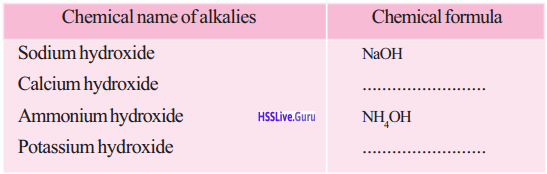Chemical name of alkalies Chemical formula Sodium hydroxide NaOH Calcium hydroxide Ca(OH)2 Ammonium hydroxide NH4OH Potassium hydroxide KOH

Question 35.
Can you find out the common factor in alkalies?
OH

Bases and alkalies:
All bases are not alkalies. Water-soluble bases are called alkalies.
NaOH and KOH are alkalies. But even though Al(OH)3 and Cu(OH). are based, they are not considered as alkalies as they are not soluble in water. Metallic oxides are generally basic in nature. But a few of them have both acidic as well as basic character. Such oxides are called amphoteric oxides. Example Al,O3, ZnO
They can react with acids as well as bases.

Question 36.
Write the equation of the dissolution of sodium hydroxide in water.
NaOH → Na+ +OH (hydoxide ion)

Question 37.
Complete the given equation of the ionization of calcium hydroxide.
Ca(OH)2 → Ca+2 +……….
Ca(OH)2→ Ca+2 + 2OH

Question 38.
Which is the common ion released when alkalies dissolve in water?
OH-
Alkalies are substances which can increase the concentration of hydroxide (OH) ions in anaqueous solution.

Question 39.
Write common names of some alkalies, their chemical names and chemical formulae

Question 40.
The equations representing the ionization of some acids and alkalies are given below. Fill in the blanks.
HCl → H+ + Cl
KOH → K+ +OH
H2CO3 → 2H+ + CO32-
NH4OH → NH+4 + OH
HNO3 → H+ + NO3Question 41.
What is Arrhenius theory?
According to Arrhenius theory, acids are substances which liberate H+ ions in aqueous solution and bases are substances which liberate OH- ions in aqueous solutions.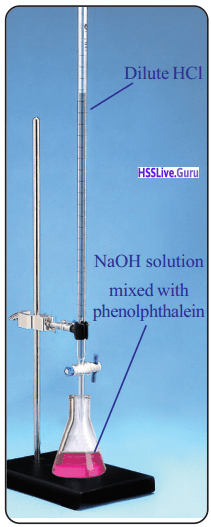Question 42.
What was the color of NaOH solution when phenolphthalein was added?
Pink

Question 43.
Which nature of the NaOH solution is indicated here?
Alkaline

Question 44.
What do you infer from the decrease in the intensity of colour of the NaOH solution on adding HCl?
Concentration of NaOH is decreasing

Question 45.
When the color disappears completely will there be any NaOH left behind in the conical flask?
No

Question 46.
Add a few drops of NaOH solution to the completely decolorized. What change can you observe? What will be the reason?
Pink color reappears concentration of NaOH in-creases.

Question 47.
To this again add dilute HCl drop by drop and stir it. What do you observe?
Pink color disappears

Question 48.
What are neutralization reactions?
Acid and alkali react with each other to nullify their individual properties. Such chemical reactions are called neutralization reactions.

Question 49.
Write the chemical equation for the neutralization reaction between sodium hydroxide and dilute hydrochloric acid.
NaOH + HCI → NaCI + H2O

Question 50.
You have already recorded the volume of HCI used for neutralization of 20 mL NaOH in the earlier experiment. Change the concentration of the acid and repeat the experiment. Is there any change in the volume of HCI?
Yes

Question 51.
Can you find out more examples for neutralization reaction?

• Slaked lime is sprinkled in farms to reduce acidity
• Antacids are used to remove acidity in the stom¬ach.

Question 52.
What happens when the acid level in the stomach is high?
It increases the acidity of the stomach

Question 53.
What do we do in such situations?
We take antacids.

Question 54.
What are antacids?
The medicines used for reducing acidity in the stomach are known as antacids.

Question 55.
What types of substances are present in antacids?
Alkaline

Question 56.
How do they work?
They neutralize acid in the stomach.

Question 57.
Take equal quantities of dilute hydrochloric acid, sodium hydroxide solution and distilled water in three test tubes. Use red litmus paper and blue litmus paper to find out the nature of the solutions. Also, add two or three drops of phenolphthalein solution to the three test tubes. Record the observation and find out the nature of the substances.
Do you notice any color change in distilled water? What property of water is revealed here?
No’, water is neutral

Question 58.
What happens to the amount of H+ ions when a little acid is added to water?
Increase

Question 59.
What happens if alkali is added?
Amount OH increases

Question 60.
What is pH value?
Determination of pH value is the scientific method for finding the acidic/alkaline nature of substances. The Danish scientist Sorensen devised the pH scale for this. The pH scale was devised based on the H+ ion concentration in the solution.

Question 61.
Observe the pH scale and answer the questions given below. What is the pH value of the neutral solution?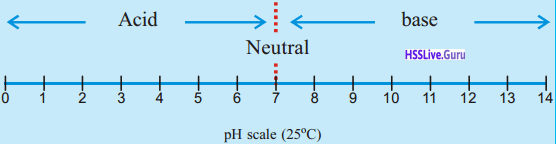7

Question 62.
What is the nature of the solution having pH value more than 7?
Basic

Question 63.
What is the nature of the solution having pH value less than 7?
Acidic

Question 64.
What is pH scale?
The pH scale is the method used to express the acidic /basic nature of a substance based on the amount of H ions present in their aqueous solutions.
On the basis of the pH scale, the pH value of a neutral solution is 7. The pH value of acids is less than

Question 65.
How the pH value can be find out using pH solution?
Add a drop of pH solution to the solution whose pH is to be determined or dip the pH paper into it. The pH value of the solution can be determined by comparing with the pH color chart.

Question 66.
Find the pH value of the following substances using the pH paper and complete the table given below.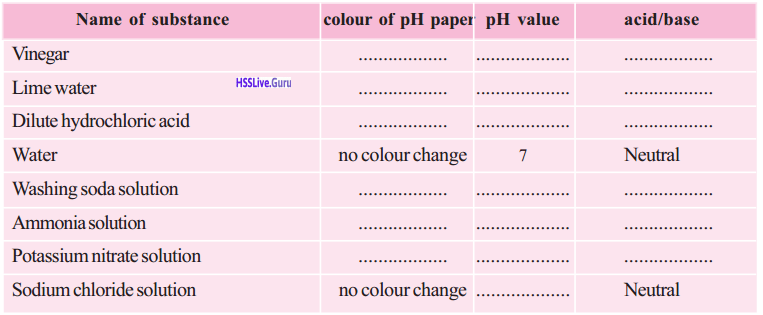Name of Substance colour of pH paper pH value acid/ base Vinegar Red less than 7 Acid Lime water Blue more than 7 base dil. Hydro­chloric acid Red less than 7 Acid Water no colour change 7 neutral Washing soda Solution Blue more than 7 base Ammonia Solution Blue more than 7 base Potassium nitrate solution no colour change 7 neutral Sodium chloride solution no colour change 7 neutral

Question 67.
It is better to determine the pH of soil before farming? Why?
The pH of soil is an important factor for crops. It is important to identify whether the soil of a region is suitable for a particular crop. Acidic soil is suitable for some crops while basic soil for a few others.

Question 68.
What are the products of the reaction between dilute hydrochloric acid and sodium hydroxide solution?Question 69.
Name the products formed when the common component of an acid and the common component of an alkali combine together.
H2O (water)Question 70.
Which is the positive ion present in sodium hydroxide? Which is the negative ion present in hydrochloric acid?
Na+ Cl

Question 71.
Write the chemical formula of the compound formed by the combination of these two ions. Identify this substance.
Na+ + Cl → NaCI

Question 72.
What is naturalization?
Neutralization is the process in which acid and alkali react with each other to form salt and water. Acid + Alkali → Salt + water

Question 73.
Complete the equation for the reaction between dilute sulphuric acid (H2S04) and magnesium hydrox¬ide Mg(OH)2.
Mg(OH)2 + H2SO4 → + 2H2O
Mg(OH)2 + H2SO4 MgSO4 + 2H2O

Question 74.
What are the products formed?
Magnesium sulfate (MgSO4) and water (H2O)

Question 75.
Identify the salts given in the table and find out the acids and alkalies required for the formation of saltSalt Chemical formula Acid Alkali Magnesium chloride MgCl2 HCI Mg(OH)2 Calcium sulphate CaSO4 H2SO4 Ca(OH)2 Aluminium sulphate Al2(SO4)3 H2SO4 Al(OH)3 Sodium nitrate NaNO3 HNO3 NaOH Potassuim ‘ phosphate K3PO4 H3PO4 KOH

Question 76.
Write the name and symbol of some positive ions and negative ions.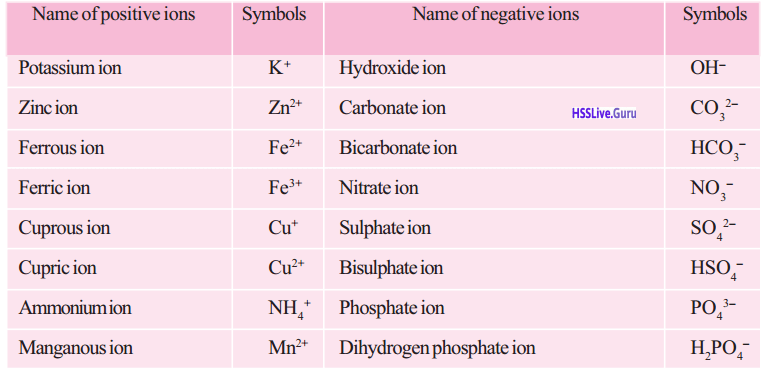Question 77.
Write the name and symbol of some positive ions and negative ions.
Formula — Name
KOH — Potassium hydroxide
K2CO3 — Potassium carbonate
KHCO3 — Potassium bicarbonate
KNO3 — Potassium nitrate
K2SO4 — Potassium sulphate
KHSO4 — Potassium bisulphate
K3PO4 — Potassium phosphate
KH2PO4 — Potassium dihydrogen phosphate
Zn(OH)2 — Zinc hydroxide
ZnCO3 — Zinc carbonate
Zn(HCO3)2 — Zinc bicarbonate
Zn(NO3)2 — Zinc nitrate
ZnSO4 — Zinc sulfate
Zn(HSO4)2 — Zinc bisulfate
Zn3(PO4)2 — Zinc phosphate
Zn(H2PO4)2 — Zincdihydrogen phosphate
Fe(OH)2 — Ferrous hydroxide
FeCO3 — Ferrous carbonate
Fe(HCO3)2 — Ferrous bicarbonate
Fe(NO3)2 — Ferrous nitrate
FeSO4 — Ferrous sulfate
Fe(HSO4)3 — Ferrous bisulfate
Fe3(PO4)2 — Ferrous phosphate
Fe(H2PO4)2 — Ferrous dihydrogen phosphate
Fe(OH)3 — Ferric hydroxide
Fe2(CO3)3 — Ferric carbonate
Fe(HCO3)3 — Ferric bicarbonate
Fe(NO3)3 — Ferric nitrate
Fe2(SO4)3 — Ferric sulphate
Fe(HSO4)3 — Ferric bisulphate
FePO4 — Ferric phosphate
Fe(H2PO4)3 — Ferric dihydrogen phosphate
CuOH — Cuprous hydroxide
Cu2CO3 — Cuprous carbonate
CuHCO3 — Cuprous bicarbonate
CuNO3 — Cuprous nitrate
Cu2SO4 — Cuprous sulphate
CuHSO4 — Cuprous bisulphate
Cu3PO4 — Cuprous phosphate
CuH2PO4 — Cuprous dihydrogen phosphate
Cu(OH)2 — Cupric hydroxide
CuCO3 — Cupric carbonate
Cu(HCO3)2 — Cupric bicarbonate
Cu(NO3)2 — Cupric nitrate
CuSO4 — Cupric sulphate
Cu(HSO4)2 — Cupric bisulphate
Cu3(PO4)2 — Cupric phosphate
Cu(H3PO4)2 — Cupric dihydrogen phosphate
NHOH — Ammonium hydroxide
(NH4)2CO3 — Ammonium carbonate
NH4HCO3 — Ammonium bicarbonate
NH4NO3 — Ammonium nitrate
(NH4)2SO4 — Ammonium sulphate
NH4HSO4 — Ammonium bisulfate
(NH4)3PO4 — Ammonium phosphate
NH4H2PO4 — Ammonium dihydrogen phosphate
Mn(OH)2 — Manganous hydroxide
MnCO3 — Manganous carbonate
Mn(HCO3)2 — Manganous bicarbonate
Mn(NO3)2 — Manganous nitrate
MnSO4 — Manganous sulfate
Mn(HSO4)2 — Manganous bisulfate
Mn3(PO4)2 — Manganous phosphate
Mn(H2PO3)3 — Manganous dihydrogen phosphate

Question 78.
The name of few salts and their chemical formulae are given in the table. Complete the table adding names of more salts and write the positive ions and negative ions.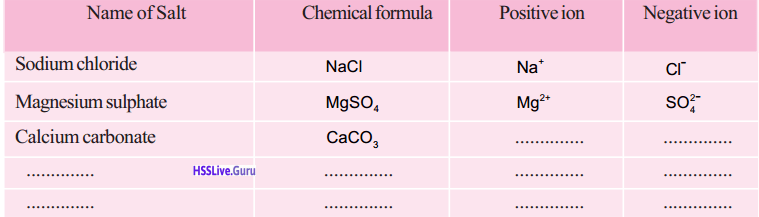Nameof salt Chemical formula Positive ion Negative ion Sodium Chloride NaCl Na+ Cl– Magnesium sulphate MgSO4 Mg2+ SO42– Calcium carbonate CaCO3 Ca2+ CO32– Sodium nitrate NaNO3 Na+ NO5 Aluminium sulphate Al2(SO4)3 Al3+ SO42–

Question 79.
What is the number of positive ions and negative ions in a ‘molecule’ of NaCI?
Anaswer:
Positive ions 1, Negative ions 1

Question 80.
What would be the sum of the charges of the posi¬tive ions and negative ions in a ‘molecule’ of NaCI?
1+ + 1 = 0 (zero)

Question 81.
Why slats are neutral in nature?
Salts are electrically neutral. The sum of the charge of the positive ions and negative ions in a salt will be zero.

Question 82.
What are the method of writing chemical formulae of salts?

• While writing the chemical formula, first write the symbol of the positive ion and then the symbol of the negative ion.
• Write the numbers indicating the charge of each ion as subscripts after interchanging them.
• Simplify the subscripts and write them in the smallest whole-number ratio.

Question 83.
Write the different stages in writing the chemical formulae of compounds formed by the combination of magnesium ion (Mg3+) with phosphate ion (PO43-) and carbonate ion (CO32-)
Mg2+ PO43
Mg2+CO32
Mg3 (PO4)2
Mg2/2 (CO3)2/2
MgCO3Question 84.
Some positive ions and negative ions are given in the table. Write the name and chemical formulae of the maximum possible salts formed by them.
Positive ion — Negative ion
Ca2+ (calcium ion) — Cl (chloride ion)
NH4+ (Ammonium ion) — SO42– (sulphate ion)
PO43– (Phosphate ion)
1) Calcium chloride CaCl2?
2) Calcium sulphate CaSO4
3) Calcium phosphate Ca3(PO4)2
4) Ammonium chloride NH4Cl
5) Ammonium sulphate (NH4)2SO4
6) Ammonium phosphate (NH4)3PO4

Question 85.
Give example for some salts that are used as fertilizers.

• Ammonium sulphate.(NH4)2SO4
• Potassium chloride KCl
• Sodium nitrate NaNO3

Question 86.
We use various salts in our daily life. A list of some of these salts and their chemical formulae are given in the table. Analyze the table and complete.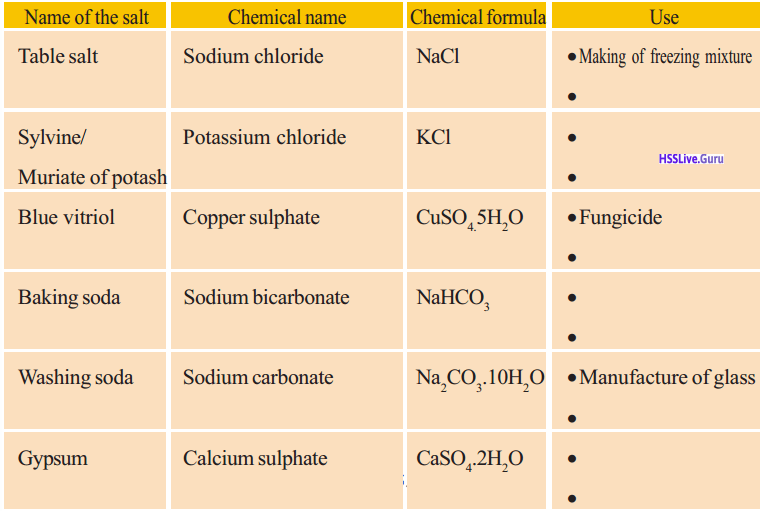Let Us Assess

Question 1.
Complete the chemical equations for the following ionization equation.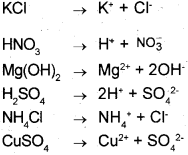Question 2.
Identify the symbol from the box and write against their names.
SO32– , NO3 , HCO3, OH, CO32-, HSO4
Carbonate —
Bisulphate —
Sulfite —
Nitrate —
Hydroxide —
Bicarbonate —
Carbonate → CO32
Bisulphate → HSO3
Sulfite → SO32
Nitrate → NO3
Hydroxide → OH
Bicarbonate → HCO3

Question 3.
a) Name the salt formed by the reaction between
magnesium hydroxide [Mg(OH)2] and dil. hydrochloric acid (HCl).
b) Write the equation for the reaction
c) Which is the acid required for the preparation of magnesium sulfate?
a) Magnesium Chloride
b) Mg(OH)2 + 2HCl MgCl2 + 2H2O
c) H2SO4 (sulphuric acid)

Question 4.
List the cation and anions of the substance given in the table.Compound Chemical formula Cation Anion Potasium Chloride KCl K+ Cl– Magnesium Chloride MgCl2 Mg2+ Cl– Sodium Nitrate NaNO3 Na+ NO3– Ammonium Chloride NHCl4 NH4+ Cl– Aluminium Sulphate Al2(SO4)3 Al3+ C042– Calcium Phosphate Ca(PO4)3 Ca2+ PO43–

Question 5.
A little distilled water is taken in a beaker.
A. What is the pH value of the distilled water?
B. What happens to the pH value when the following substances are added to the water? Justify your answer.
i) Caustic soda
ii) Vinegar
A. Seven
B. i) pH value increases, solution becomes basic
ii) pH value decreases, solution becomes acidic

Question 6.
Match the column A, B, and C by identifying the correct chemical formulae and the use of the salts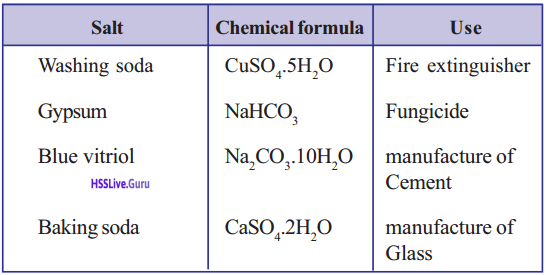Salt Chemical Use Washing soda Na2CO3.10H2C Manufacture of Glass Gypsum CaSO4.2H2O Manufacture of cement Blue vitriol CuSO4.5H2O Fungicide Baking soda NaHCO3 Fire extinguisher

Question 7.
The pH values of some substances are given in the table. Analyze the table and answer the questions

 Substance pH value Vinegar 4.2 Limewater 10.5 Milk 6.4 Water 7 Toothpaste 8,7 Blood 7.36

a. Is blood acidic or basic in nature?
b. The pH value of pure milk is 6.4. Does the pH value increase or decrease when milk changes . to curd. Justify your answer
c. Among the substances given in the above table
(i) Which one is strongly basic?
(ii) Which one has weak acidic nature
a) Basic
b) pH value decrease. Curd is lactic acid
c) i) Limewater
ii) Blood

Extended Activities

Question 1.
Organic acids are present in a number of substances we use in our daily life.
(eg. Tomato, orange, apple, grapes, curd, etc.) Identify the organic acids in each of them and tabulate.
Tomato — oxalic acid
Orange — Citric acid
Apple — Malic acid
Grapes — Tartaric acid
Curd — Lactic acid, Butiric acid

Question 2.
Haven’t you conducted an activity to find the pH value of the soil related to different crops? Identify the pH values of soil samples collected from different places.
Prepare a list of the crops that are suitable for the soil of each area on the basis of its pH value

 Name of crop Suitable pH Paddy 5-8 Coconut 5-8 Rubber 5-6.5 Tea 4.0-6.5 Coffee 4.5-5.5 Potato 5.2-7.5 Cocoa 5.5-7.0 Tobacco 5.5-6.00 Brinjal 5.5-6.50 Cucumber 6.0-7.5 Bitter gourd 6.0-7.5 Watermelon 6.5-70 Tapioca 5.5-7.0

Question 3.
a) Complete the equations of the ionization of phosphoric acid.
H3PO4 → H+ + H2PO4 (Dihydrogenphosphate ion)
H3PO4 → H+ + …………. (Hydrogenphosphate ion)
…….. → H+ + PO43- (phosphate ion)
b) How many types of salts can be formed by phosphoric acid? Why?
c) Write the chemical name of the following salts.
Mg(H2PO4)2
MgHPO4
Mg3(PO4)2
a) H2PO4 → H+ + HPO42- (Hydrogenphosphate ion)
HPO42- → H + PO43- (phosphate ion)
b) It is tribasic
c) Magnessium dihydrogen phosphate Magnessium hydrogenphosphate Magnessium phosphate

Question 4.
Solution of sodium carbonate, potassium chloride, and ammonium sulfate are taken in separate beakers. Dip a litmus paper (red, blue) in each beaker.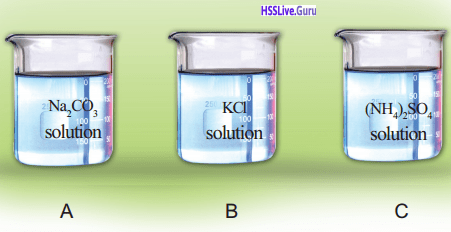Salt Colour of the litmus paper Nature of the substance A Red litmus turns Basic to blue B No color change Neutral C Blue litmus turns to red Acidic

i) Observe the color change of litmus paper and tabulate.
ii) Name the acid and alkali that react to form each salt given above?
iii) Can you explain the color change of the litmus paper on the basis of the nature of the acid and alkali that react to form the salt?
(hint: Potassium chloride is a salt formed by the reaction between a strong acid and a strong alkali)

i) Salts formed by the reaction between a strong acid and a strong base will be neutral in nature, eg: KCI
ii) Salts formed by the reaction between a strong base and weak acid will be slightly basic eg: Na2CO3
iii) Salts formed by the reaction between a weak base and strong acid will be slightly acidic eg:(NH4)2SO4

### Acids, Bases, Salts More Questions and Answers

Question 1.
Non-metalic oxides like CO2, SO2, and NO2 shows acidic nature, justify it.
Compounds formed by the reaction of the above nonmetal oxide dissolves in water shows acidic character.

Question 2.
Take quick lime (Calcium oxide) in a beaker containing water. Take a little of this in a test tube and show a red litmus paper.
Red litmus paper turns to blue because calcium oxide dissolves in water to form calcium hydroxide. It is basic in nature.

Question 3.
Complete the equation of the reaction between calcium oxide and water.
CaO + H2O → Ca(OH)2Question 4.
What can be inferred about the nature of this substance from the litmus test?
Alkaline nature

Question 5.
Classify the following oxides into acidic oxide and basic oxide
SO3, NO2, CaO, K2O P2O5, Na2O, CO2, MgO

 Acidic Oxide Basic Oxide SO3 Cao NO2 K2O P2O5 Na2O CO2 MgO

Question 6.
Try to write the chemical equation for the reaction of Fe with hydrochloric acid.
Fe + 2 HCI → FeCl2 + H2

Question 7.
Analyze the chemical equation for the reactions taking place when hydrochloric acid dissolves in water.
HCl → H+ + Cl
H+ + H2O → H3O+ (Hydronium ion)

Question 8.
Explain the basis of classification of acid into monobasic, Dibasic and tribasic acids.
An acid molecule dissolves in water to liberate H+ ions. Based on this acids are classified into monobasic, Dibasic and tribasic acids. HCl is a monobasic acid.

Question 9.
Explain the dissociation of H2SO4
H2SO4 → H+ + HSO4+ (Bisulphate ion)
HSO4– → H+ + SO42+ (Sulphate ion)

Question 10.
Classify the following acids into monobasic, dibasic and tribasic acids,
H2CO3, HNO3, H3PO4, H2SO4, HCl, H2S4

Question 11.
During the rainy season, the farmers are using slaked lime in agricultural fields, give reason.
To reduce the acidity of the soil.

Question 12.
Write the chemical formula of slaked lime.
Calcium hydroxide – Ca(OH)4

Question 13.
Most of the alkalies are also known by the common names in addition to their chemical names. Caustic soda, milk of lime, milk of magnesia and caustic potash are the common names of some alkalies. Identify the chemical formula with the help of the given table.

Question 14.
Observe the chemical equation of the dissolution of Sodium hydroxide in water.
NaOH → Na+ + OH (Hydroxide ion)
Now complete the given chemical equation for the ionization of Calcium hydroxide.
Ca(OH)2 → + ………. + …………..
Ca(OH)2 → Ca+ + 2OH

Question 15.
Which is the common ion released when alkalies dissolved in water?
OH

Question 16.
Difference between bases and alkalies?
Bases are compounds that are opposite in acids in their characteristics.
Bases dissolving in water are alkalies. The metal hydroxide which dissolves in water functioning as strong alkalies.
Sodium hydroxide, Potassium hydroxide are alkalies, but Al(OH)3, Ca(OH)2 are bases.

Question 17.
What is meant by a neutralization reaction?
An acid combines with alkali to nullify their individual properties. Such reactions are called neutralization reaction.

Question 18.
Give equation for the neutralization reaction of Sodium hydroxide with Hydrochloric acid.
NaOH + HCl → NaCl + H2O

Question 19.
If we add small quantity of acid to water, what happens to the quantity of H+ ion?
Quantity of H+ ion increases.

Question 20.
What change occurs when alkali is added?
Quantity of OH+ ion increases.

Question 21.
Complete the table and find the pH value by using pH paper of the following substances.

Question 22.
If the pH value increases whether acid or alkali character increases?
Alkali character increases

Question 23.
If the pH value increases whether the H+ ion concentration decreases or increases?
Decreases

Question 24.
The names of a few salts and their chemical formulas are given below. Find the cations & anions and complete the table.

Question 25.
Can you find out the acid and alkali responsible for the formation of the salts given in the table from their chemical formula

Question 26.
Prepare a table by writing the chemical formula of more salts that you are familiar with and also the names of the acid and alkalies from which they are formed.

Question 27.
Find out how the chemical formula of the salts are written analyzing the table.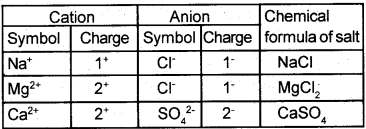Question 28.
Give the salts used as chemical fertilizers?
Ammonium Sulphate – (NH4)2SO4
Potassium Chloride – KCl
Sodium Nitrate – NaNO3

Question 29.
a) Complete the chemical equation for the ionization of Phosphoric acid.
H3PO4 → H+ + H2PO4+
H3PO4+ → H+ + ……….
………. → H+ + PO43-
b) Based on the ionization equation given above Phosphoric acid is a tribasic acid? Justify it.
a) H3PO4 → H+ + H2PO42-(Dihydrogen Phosphate ion)
H2PO4 → H+ + HPO42- (Hydrogen Phosphate ion)
HPO42- → H+ + PO43- (Phosphate ion)
b) In Phosphoric acid in aqueous solution number of H+ ion release is 3. Hence it is a tribasic acid.

Question 30.
Two solutions A and B having pH value 5 and 9, respectively. Which one shows acidic character and which one shows alkaline character. Why?
A shows acidic character because the pH value is below 7, B shows alkaline character because the pH value is above 7.

Question 31.
List the cations and anions of the substances given in the table.Substance Chemical formula Anion Cation Potassium Sulphate K2SO4 SO42– Mg2+ Ammonium Nitrate NH4NO3 NO31- NH4+ Calcium Chloride CaCl2 Cl– Ca2+ Magnesium Carbonate MgCO3 CO32– Mg2+

Question 32.
Some cations and anions are given. Write the chemical formula of all the salts possible by combining them.